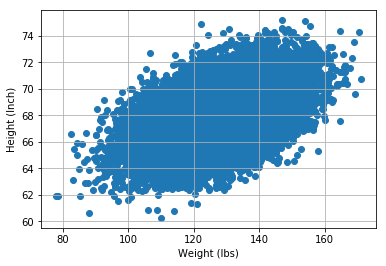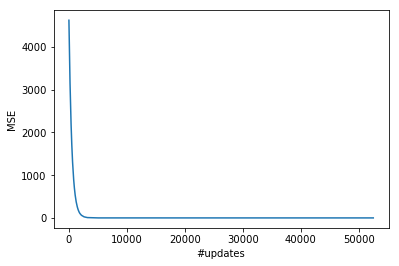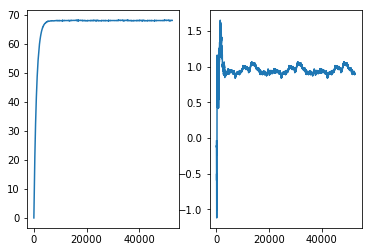## mlcourse.ai – Open Machine Learning Course¶

Author: Yury Kashnitskiy. Translated by Sergey Oreshkov. This material is subject to the terms and conditions of the Creative Commons CC BY-NC-SA 4.0 license. Free use is permitted for any non-commercial purpose.

# Assignment #8 (demo). Solution

## Implementation of online regressor

Same assignment as a Kaggle Kernel + solution.

Here we'll implement a regressor trained with stochastic gradient descent (SGD). Fill in the missing code. If you do evething right, you'll pass a simple embedded test.

## Linear regression and Stochastic Gradient Descent

In :
import numpy as np
import pandas as pd
from tqdm import tqdm
from sklearn.base import BaseEstimator
from sklearn.metrics import mean_squared_error, log_loss, roc_auc_score
from sklearn.model_selection import train_test_split
%matplotlib inline
from matplotlib import pyplot as plt
import seaborn as sns
from sklearn.preprocessing import StandardScaler


Implement class SGDRegressor. Specification:

• class is inherited from sklearn.base.BaseEstimator
• constructor takes parameters eta – gradient step ($10^{-3}$ by default) and n_epochs – dataset pass count (3 by default)
• constructor also creates mse_ and weights_ lists in order to track mean squared error and weight vector during gradient descent iterations
• Class has fit and predict methods
• The fit method takes matrix X and vector y (numpy.array objects) as parameters, appends column of ones to X on the left side, initializes weight vector w with zeros and then makes n_epochs iterations of weight updates (you may refer to this article for details), and for every iteration logs mean squared error and weight vector w in corresponding lists we created in the constructor.
• Additionally the fit method will create w_ variable to store weights which produce minimal mean squared error
• The fit method returns current instance of the SGDRegressor class, i.e. self
• The predict method takes X matrix, adds column of ones to the left side and returns prediction vector, using weight vector w_, created by the fit method.
In :
class SGDRegressor(BaseEstimator):

def __init__(self, eta=1e-3, n_epochs=3):
self.eta = eta
self.n_epochs = n_epochs
self.mse_ = []
self.weights_ = []

def fit(self, X, y):
X = np.hstack([np.ones([X.shape, 1]), X])

w = np.zeros(X.shape)

for it in tqdm(range(self.n_epochs)):
for i in range(X.shape):

new_w = w.copy()
new_w += self.eta * (y[i] - w.dot(X[i, :]))
for j in range(1, X.shape):
new_w[j] += self.eta * (y[i] - w.dot(X[i, :])) * X[i, j]
w = new_w.copy()

self.weights_.append(w)
self.mse_.append(mean_squared_error(y, X.dot(w)))

self.w_ = self.weights_[np.argmin(self.mse_)]

return self

def predict(self, X):
X = np.hstack([np.ones([X.shape, 1]), X])

return X.dot(self.w_)


Let's test out the algorithm on height/weight data. We will predict heights (in inches) based on weights (in lbs).

In :
data_demo = pd.read_csv('../../data/weights_heights.csv')

In :
plt.scatter(data_demo['Weight'], data_demo['Height']);
plt.xlabel('Weight (lbs)')
plt.ylabel('Height (Inch)')
plt.grid();In :
X, y = data_demo['Weight'].values, data_demo['Height'].values


Perform train/test split and scale data.

In :
X_train, X_valid, y_train, y_valid = train_test_split(X, y,
test_size=0.3,
random_state=17)

In :
scaler = StandardScaler()
X_train_scaled = scaler.fit_transform(X_train.reshape([-1, 1]))
X_valid_scaled = scaler.transform(X_valid.reshape([-1, 1]))


Train created SGDRegressor with (X_train_scaled, y_train) data. Leave default parameter values for now.

In :
# you code here
sgd_reg = SGDRegressor()
sgd_reg.fit(X_train_scaled, y_train)

100%|██████████| 3/3 [00:16<00:00,  5.68s/it]

Out:
SGDRegressor(eta=0.001, n_epochs=3)

Draw a chart with training process – dependency of mean squared error from the i-th SGD iteration number.

In :
# you code here
plt.plot(range(len(sgd_reg.mse_)), sgd_reg.mse_)
plt.ylabel('MSE');Print the minimal value of mean squared error and the best weights vector.

In :
# you code here
np.min(sgd_reg.mse_), sgd_reg.w_

Out:
(2.7151352406643627, array([67.9898497 ,  0.94447605]))

Draw chart of model weights ($w_0$ and $w_1$) behavior during training.

In :
# you code here
plt.subplot(121)
plt.plot(range(len(sgd_reg.weights_)),
[w for w in sgd_reg.weights_]);
plt.subplot(122)
plt.plot(range(len(sgd_reg.weights_)),
[w for w in sgd_reg.weights_]);Make a prediction for hold-out set (X_valid_scaled, y_valid) and check MSE value.

In :
# you code here
sgd_holdout_mse = mean_squared_error(y_valid,
sgd_reg.predict(X_valid_scaled))
sgd_holdout_mse

Out:
2.6708681207033784

Do the same thing for LinearRegression class from sklearn.linear_model. Evaluate MSE for hold-out set.

In :
# you code here
from sklearn.linear_model import LinearRegression
lm = LinearRegression().fit(X_train_scaled, y_train)
print(lm.coef_, lm.intercept_)
linreg_holdout_mse = mean_squared_error(y_valid,
lm.predict(X_valid_scaled))
linreg_holdout_mse

[0.94537278] 67.98930834742858

Out:
2.670830767667634
In :
try:
assert (sgd_holdout_mse - linreg_holdout_mse) < 1e-4
print('Correct!')
except AssertionError:
print("Something's not good.\n Linreg's holdout MSE: {}"
"\n SGD's holdout MSE: {}".format(linreg_holdout_mse,
sgd_holdout_mse))

Correct!